• 本文介绍高斯核密度估计和Ep核密度估计两种核模型算法： 1、 Epanechnikov 核函数介绍 核密度估计是在概率论中用来估计未知的密度函数，属于非参数检验方法之一，由Rosenblatt (1955)和Emanuel Parzen(1962)...
目标检测，也叫目标提取，是一种基于目标几何和统计特征的图像分割，它将目标的分割和识别合二为一，其准确性和实时性是整个系统的一项重要能力。尤其是在复杂场景中，需要对多个目标进行实时处理时，目标自动提取和识别就显得特别重要。

随着计算机技术的发展和计算机视觉原理的广泛应用，利用计算机图像处理技术对目标进行实时跟踪研究越来越热门，对目标进行动态实时跟踪定位在智能化交通系统、智能监控系统、军事目标检测及医学导航手术中手术器械定位等方面具有广泛的应用价值。

本文介绍高斯核密度估计和Ep核密度估计两种核模型算法：

1、Epanechnikov核函数介绍

核密度估计是在概率论中用来估计未知的密度函数，属于非参数检验方法之一，由Rosenblatt (1955)和Emanuel Parzen(1962)提出，又名Parzen窗（Parzen window）。

假设我们有n个数X1-Xn,我们要计算某一个数X的概率密度有多大。核密度估计的方法是这样的：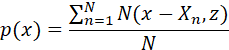(1)

其中N(x,z)为正态分布的概率密度函数,z为设定的参数。

核密度估计算法的基本原理是在对某一事物的概率分布的情况下，假设一个数在观察的过程中出现了，就可以假定这个数相对应的概率密度比较大，从而可以得出和这个数相邻的数的概率密度也会比较大，反之，离这个数较远的数的概率密度比较小。基于这一原理，我们可以通过一个函数来映射出每个数概率密度之间的关系。

假设xi,i=1,2,…N为同一未知概率密度函数产生的维度为d的N个样本点。核密度估计函数表示如下：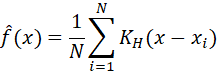(2)

其中KH为核函数，可以表示为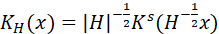。H为d×d大小的正定带宽矩阵。当简化带宽矩阵为单参数h时，(2)式变为我们所熟悉的形式：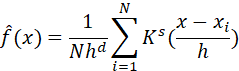(3)

多维变量的核函数Ks可以通过每个维度下单变量核函数Kj的乘积计算来表示：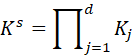(4)

xi∈{x1,x2,…,xN}为图像中位于坐标(x0,y0)的像素点在第i时刻的色彩向量，{x1,x2,…,xN}为当前像素样本集Sa。对于t时刻此像素点的观测值xt而言，其密度函数p(xt)的核密度估计则可定义如下：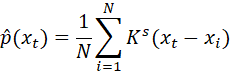(5)

其中，Ks为选择的核函数，满足性质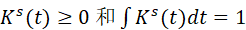。

所采集的图像数据一般为多通道的彩色图像(例如RGB三通道)，利用公式(2)、(3)，可以写出d维颜色通道的核函数乘积：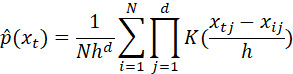(6)

单维度Epanechnikov函数表达式如下：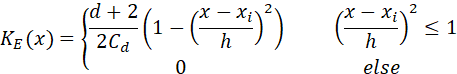(7)

Cd表示d维超球体体积，以RGB图像为例，C3是半径为1的球的体积，即C3=4π∙13/3。此时像素的输入xt=xt1,xt2,xt3，样本点为xi=[xi1,xi2,xi3]。基于Epanechnikov核函数的三通道彩色图像像素值的概率密度估计函数为：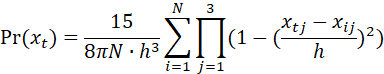(8)

2、高斯核函数介绍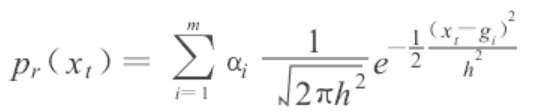展开全文• 核密度估计是非参数检验的一种，已知一列数据，估计其密度函数的过程。计算公式如下，主要包括核密度函数和带宽(窗宽)，常见的核函数为高斯函数和Epanechnikov...图2 国际贸易核密度图核密度图可以通过R、python、s...


核密度估计是非参数检验的一种，已知一列数据，估计其密度函数的过程。计算公式如下，主要包括核密度函数和带宽(窗宽)，常见的核函数为高斯函数和Epanechnikov函数，带宽则可以根据核函数求解。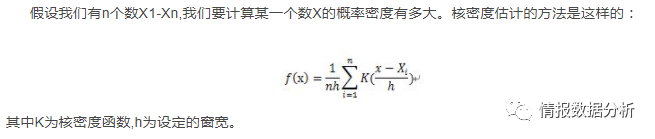图1 核密度估计原理核密度估计在经济学、金融学，以及人文地理学中较为常用，主要是用来估计金融风险、国际贸易密度、人口及GDP密度，如图2。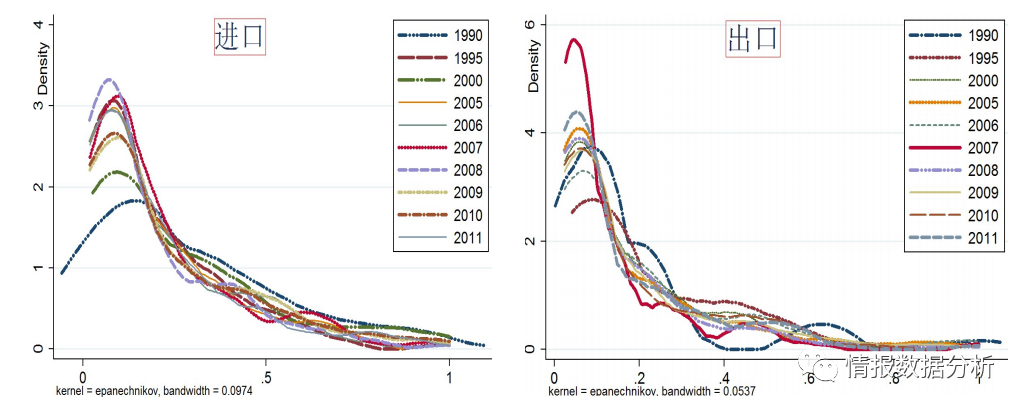图2 国际贸易核密度图核密度图可以通过R、python、stata来制作，其中stata最简单且易于上手。一年的数据很好做，但是多年的数据做到一张图上，还是难倒了不少的同学。本教程首先讲解核密度估计的原理，然后手把手教学，从数据录入stata到制作图表，再到图表的调整，15分钟你就能作出一张多年核密度图了，你的毕业论文有救啦！详细教程请点击下方的阅读原文。


展开全文• 具体什么是核密度估计建议大家去B站搜索一下，讲的很清楚，大概就是需要一个核函数，对每一个样本点进行叠加，最后再归一化的一个过程，这个过程和带宽有很大的关系（以高斯函数为例，带宽就是方差）。 我主要用...
最近需要可视化统计一个二维变量的数量分布图，网上搜了一下大概有一下两种方法：

1. 核密度估计图，通过核函数来进行密度估计

2. 六边形分箱图（Hexagonal Binning），直接计算不同bin中的点的数量

首先来说一下核密度估计图

具体什么是核密度估计建议大家去B站搜索一下，讲的很清楚，大概就是需要一个核函数，对每一个样本点进行叠加，最后再归一化的一个过程，这个过程和带宽有很大的关系。

我主要用Python的scipy.stats.gaussian_kde函数和matplotlib进行了可视化

具体代码网上很多，这里我又加了一些注释，算是给入门的看的吧：

import numpy as np
import matplotlib.pyplot as plt
from scipy.stats import gaussian_kde

x=data1#第一维数据
y=data2#第二维数据
xy = np.vstack([x,y])#将两个维度的数据进行叠加
kenal = gaussian_kde(xy)#这一步根据xy这个样本数据，在全定义域上建立了概率密度分布，所以kenal其实就是一个概率密度函数，输入对应的(x,y)坐标，就给出相应的概率密度
z = kenal.evaluate(xy)#得到我们每个样本点的概率密度
z = gaussian_kde(xy)(xy)#这行代码和上面两行是相同的意思，这行是一行的写法
idx = z.argsort() #对z值进行从小到大排序并返回索引
x, y, z = x[idx], y[idx], z[idx]#对x,y按照z的升序进行排列
#上面两行代码是为了使得z值越高的点，画在上面，不被那些z值低的点挡住，从美观的角度来说还是十分必要的
fig, ax = plt.subplots(figsize=(7,5),dpi=100)
scatter = ax.scatter(x,y,marker='o',c=z,edgecolors='none',s=25,label='label'
,cmap='Spectral_r')
cbar_ax = plt.gcf().add_axes([0.93, 0.15, 0.02, 0.7])#[left,bottom,width,height] position
cbar = fig.colorbar(scatter, cax=cbar_ax, label='Probability density')
ax.set_xlabel('xlabel')
ax.set_ylabel('ylabel')
ax.set_title('title')

下面还有两点需要注意的地方：

1. 关于带宽，函数默认采用Scott's Rule：n**(-1./(d+4))，其中n是样本量，d是纬度，也就是样本量越大，维度越低，带宽越小，毕竟样本量多了，对应的高斯函数的方差应该是需要减小的，否则就会变得非常平滑，反之，如果样本量很小，再不用较大的方差，那么也就无法进行差值了。（感觉这里和地理统计中的差值非常像，样本量小，同时方差还很低，就会出现‘牛眼’现象）。

这里我其实并不明白带宽和高斯函数标准差之间的关系，所以我自己带入高斯函数公式推导了一下：

可以看出来其实真实的高斯函数标准差是根据样本标准差（）和带宽（h）相乘得到的，所以带宽越宽，得到的核密度分布越平滑。

2. 函数的返回值是概率密度，这个概率密度对定义域进行积分其实就是1，从上面的公式也可以看出来。

至于六边形分箱图我还没有发现有多好看，好处可能是对数据统计更加准确，毕竟是一个客观统计值，没有概率这一说了，但是还是觉得KDE比较好，大家想了解的就去matplotlib官网看看吧：

https://www.osgeo.cn/matplotlib/gallery/statistics/hexbin_demo.html

https://matplotlib.org/api/_as_gen/matplotlib.pyplot.hexbin.html

展开全文python 数据可视化
• 图像及label处理为密度图并保存为csv格式 clc; clear all; seed = 95461354; rng(seed) N = 2; dataset = 'train'; path = ['I:/crowd_dataset/L/']; output_path = 'I:/crowd_dataset/L/formatted_trainval_1'; ...
图像及label处理为密度图并保存为csv格式
该方法使用matlab
427更新，解决了保存3通道图片，密度图3通道保存出错的问题
主程序
################################################
#############MERCY ROIN 2020/03/21##############
########https://blog.csdn.net/qq_42732137#######
################################################
clc; clear all;
seed = 95461354;
rng(seed)
N = 2;
dataset = 'train';
%图片链接
path = ['I:/crowd_dataset/L/'];
output_path = 'I:/crowd_dataset/L/formatted_trainval_1';
train_path_img = strcat(output_path, '/train/');
train_path_den = strcat(output_path, '/train_den/');
val_path_img = strcat(output_path, '/val/');
val_path_den = strcat(output_path, '/val_den/');
gt_path = ['I:/crowd_dataset/L/'];

img = dir('I:\crowd_dataset/L\*.jpg');%load img

mkdir(output_path)
mkdir(train_path_img);
mkdir(train_path_den);
mkdir(val_path_img);
mkdir(val_path_den);

num_images = length(img);
num_val = ceil(num_images*0.1);
indices = randperm(num_images);

for idx = 1:num_images
i = indices(idx);
imgname = img(idx).name;
matname = imgname;
csvname = imgname;
matname(end-2:end) = 'mat';
csvname(end-2:end) = 'csv';
load([gt_path matname]);%gt.mat path

if (mod(idx,10)==0)
fprintf(1,'Processing %3d/%d files\n', idx, num_images);
end

image = imread([path, imgname]);
annPoints = gt_point; %mat里函数名注意修改为自己的
%灰度处理。如果图像使用RGB
%     [h, w, c] = size(image);
if (c == 3)
im = rgb2gray(image);
end

%% gaussian kernel function
im_density = get_density_map_autogaussian(image,annPoints);%%导入自适应高斯核函数
%% data processing
for j = 1:N
%% total image 图像翻转增加数据量
im_sampled = image;
im_density_sampled = im_density(:, :, 1);
if (j==2)
im_sampled = fliplr(image);
im_density_sampled = fliplr(im_density(:, :, 1));
end

%%
%show img&den
den=255*im_density_sampled;
figure(1)
subplot(1,2,2);
imshow(den)
hold on
plot(annPoints(:,1),annPoints(:,2),'r*')
subplot(1,2,1);
imshow(im_sampled)
hold on
plot(annPoints(:,1),annPoints(:,2),'r*')

img_idx = strcat(num2str(i), '_',num2str(j));

if(idx < num_val)
imwrite(im_sampled, [val_path_img num2str(img_idx) '.jpg']);
csvwrite([val_path_den num2str(img_idx) '.csv'], im_density_sampled);
else
imwrite(im_sampled, [train_path_img num2str(img_idx) '.jpg']);
csvwrite([train_path_den num2str(img_idx) '.csv'], im_density_sampled);
end

end

end


自适应高斯核 1
%function:通过高斯核产生相应的密度矩阵
%parameter： im：输入图像，灰度图；  points：标注的点[X Y]，n*2的矩阵
function im_density = get_density_map_autogaussian(im,points)

im_density = zeros(size(im));
[h,w] = size(im_density);

if(isempty(points))
return;
end

%points为1行
if(length(points(:,1))==1)
x1 = max(1,min(w,round(points(1,1))));  %round：四舍五入，x1变成points(1,1)处的整数
y1 = max(1,min(h,round(points(1,2))));
im_density(y1,x1) = 255;
return;
end

for j = 1:length(points)
max_kernel = 65;    %最大高斯核尺寸
normal_kernel = 35; %默认高斯核尺寸
beta = 0.3;         %MCNN中给定的参数
k = 6;              %近邻数
maxpixel = 80;      %近邻的最大距离，像素

%    f_sz = normal_kernel;
%    sigma = beta * f_sz;

x = min(w,max(1,abs(double(floor(points(j,1))))));
y = min(h,max(1,abs(double(floor(points(j,2))))));
if(x > w || y > h)
continue;
end

%--------%auto adaptive 自适应高斯核--------
atemp = [];
for i = 1:length(points)
if i == j
continue;
end
xk = min(w,max(1,abs(double(floor(points(i,1))))));
yk = min(w,max(1,abs(double(floor(points(i,2))))));
if(xk > w || yk > h)
continue;
end
dis = sqrt( ((xk-x)^2  + (yk-y)^2) );
atemp = [atemp dis];
end
btemp = sort(atemp);

sum = 0;
count = 0;
if  k >= length(points)
k = length(points) - 1;
end

for m = 1:k
if btemp(m) > maxpixel
break;
end
sum = sum + btemp(m);
count = count + 1;
end

if count > 0
temp = sum/count;
else
temp = normal_kernel;
end

f_sz = double(floor(temp));
if mod(f_sz, 2) == 0
f_sz = double(f_sz + 1);
end
if f_sz > (max_kernel + 1)
f_sz = max_kernel;
end

sigma = beta * f_sz;

%    fprintf("x:%d,y:%d,f_sz:%d,sigma：%d\n",x,y,f_sz, sigma);

%--------auto adaptive 自适应高斯核定义结束--------

H = fspecial('Gaussian',[f_sz, f_sz],sigma);

%高斯核边界限定，x方向：→，y方向：↓
x1 = x - double(floor(f_sz/2)); y1 = y - double(floor(f_sz/2));   %x1左边界，y1上边界
x2 = x + double(floor(f_sz/2)); y2 = y + double(floor(f_sz/2));   %x2右边界，y2下边界
dfx1 = 0; dfy1 = 0; dfx2 = 0; dfy2 = 0;
change_H = false;
if(x1 < 1)
dfx1 = abs(x1)+1;
x1 = 1;
change_H = true;
end
if(y1 < 1)
dfy1 = abs(y1)+1;
y1 = 1;
change_H = true;
end
if(x2 > w)
dfx2 = x2 - w;
x2 = w;
change_H = true;
end
if(y2 > h)
dfy2 = y2 - h;
y2 = h;
change_H = true;
end
x1h = 1+dfx1; y1h = 1+dfy1; x2h = f_sz - dfx2; y2h = f_sz - dfy2;
if (change_H == true)
H =  fspecial('Gaussian',[double(y2h-y1h+1), double(x2h-x1h+1)],sigma);
end
im_density(y1:y2,x1:x2) = im_density(y1:y2,x1:x2) +  H;

end

end


固定核
function im_density = get_density_map_gaussian(im,points)

im_density = zeros(size(im));
[h,w] = size(im_density);

if(length(points)==0)
return;
end

if(length(points(:,1))==1)
x1 = max(1,min(w,round(points(1,1))));
y1 = max(1,min(h,round(points(1,2))));
im_density(y1,x1) = 255;
return;
end
for j = 1:length(points)
f_sz = 15;
sigma = 4.0;
H = fspecial('Gaussian',[f_sz, f_sz],sigma);
x = min(w,max(1,abs(int32(floor(points(j,1))))));
y = min(h,max(1,abs(int32(floor(points(j,2))))));
if(x > w || y > h)
continue;
end
x1 = x - int32(floor(f_sz/2)); y1 = y - int32(floor(f_sz/2));
x2 = x + int32(floor(f_sz/2)); y2 = y + int32(floor(f_sz/2));
dfx1 = 0; dfy1 = 0; dfx2 = 0; dfy2 = 0;
change_H = false;
if(x1 < 1)
dfx1 = abs(x1)+1;
x1 = 1;
change_H = true;
end
if(y1 < 1)
dfy1 = abs(y1)+1;
y1 = 1;
change_H = true;
end
if(x2 > w)
dfx2 = x2 - w;
x2 = w;
change_H = true;
end
if(y2 > h)
dfy2 = y2 - h;
y2 = h;
change_H = true;
end
x1h = 1+dfx1; y1h = 1+dfy1; x2h = f_sz - dfx2; y2h = f_sz - dfy2;
if (change_H == true)
H =  fspecial('Gaussian',[double(y2h-y1h+1), double(x2h-x1h+1)],sigma);
end
im_density(y1:y2,x1:x2) = im_density(y1:y2,x1:x2) +  H;

end

end


https://www.jianshu.com/p/935a74d83a50 ↩︎


展开全文计算机视觉 matlab 机器学习
• %function:通过高斯核产生相应的密度矩阵 %parameter： im：输入图像，灰度； points：标注的点[X Y]，n*2的矩阵 function im_density = get_density_map_autogaussian(im,points) im_density...
• 用matlab实现对6个样本点实现直方估计、高斯核密度估计
• Matlab自带很多有意思的彩蛋命令，包括二维...3、peaks峰值函数，本质是二元高斯分布的概率密度函数。4、image倒过来的小孩。5、spy小狗。spy是用来可视化稀疏矩阵的。6、knot绳结。7、cruller8、xpklein克莱因...
• 一、一元高斯分布概率密度 均值为0，方差为1的标准一元正态分布，概率密度函数为：在均值为 ，方差为 的情况下，需要标准化一下: ，标准化之后方差变为1，标准化的意义在于将数据点 到均值 的距离转化为数据点 到...
• 核密度估计（KDE）完全没有上述的问题。 构建KDE需要准备核函数：下面是常用的核函数图形和定义。 构建一个KDE包含两部分： ①把kernel偏移到特定的位置 ②设定带宽bindwidth 下高斯k...
• 参考： ...人群基数方法分类 （1） 基于目标检测的方法： 这类方法就是通过对图像上每个行人或这人头进行定位与识别，再根绝结果...优点在于可以做到准确的行人或者人头位置，但缺点在于对高密度的人群图像来说，其检...
• 提出适用于高密度人群的自适应高斯核，用于对图像进行预处理操作，可以自适应地对图像进行去噪处理，相比于传统的高斯滤波器获得更好的平滑效果。
• 所以有必要铺垫一个很重要的概率密度函数，高斯分布。既然是分布，它本身就代表一个概率密度函数。只不过高斯分布，拆解开来，就是服从某种特点的概率密度函数。我们知道高斯分布的图形是这样的:这个图像是高尔顿最...
• 该方法结合了单高斯模型和核密度估计模型进行初始背景建模，利用背景差分图像，过滤掉非动态背景区域，对动态背景区域采用核密度估计进行像素分类。同时，对非动态背景区域，采用渐进式更新；对动态背景区域，采用非...
• 最近在做人群密度估计，是一种以密度图的形式表示人群密集程度的方法，大概如下图左图是某公共场所的人群图，右图是人群密度图，也就是用来训练生成人群密度图网络的标签。首先要将原图中左右人头的坐标标定出来，...
• 我需要代码来做二维核密度估计(KDE),我发现SciPy实现太慢了.所以,我已经编写了一个基于FFT的实现,但有些事情让我很困惑. (FFT实现还强制执行周期性边界条件,这就是我想要的.)该实现基于从样本创建简单的直方,然后...
• 最近在做人群密度估计，是一种以密度图的形式表示人群密集程度的方法，大概如下图左图是某公共场所的人群图，右图是人群密度图，也就是用来训练生成人群密度图网络的标签。首先要将原图中左右人头的坐标标定出来，...
• 假设图像中的人头大小是固定的，例如MCNN中使用几何自适应高斯核来估计图像中每个人头的大小并转换为密度图密度图中各个人头区域的概率之和为1，得到完整的人群图像密度图后，对其进行积分则是人群数目； 经典...机器学习
• 来获得以下绘图：(MWE在问题的底部)很明显，这些参数之间有一个关系，因为一个是高斯滤波器，另一个是高斯核密度估计。在每个参数的定义是：sigma : scalar or sequence of scalars Standard deviation for G...
• 高斯核 举个例子：假设中心点的坐标为（0,0），那么取距离它最近的8个坐标点，假设∂=1.5， 这个时候还要确保这9个数和为1，这9个点的总权重为0.1787147，因此上面9个值每一个值除以0.4787147 得到最
• 高斯噪声就是它的概率密度函数服从高斯分布（即正态分布）的一类噪声。其在图像当中常表现为能引起较强视觉效果的孤立像素点或像素块。噪声的出现会给图像带来干扰，让图像变得不清楚。 高斯滤波就是对整幅图像进行...
• 基本概念 二维高斯模糊，或者说高斯滤波，是图像处理中非常常见的操作。操作的核心是使用一个从高斯分布中采样...由于高斯分布的概率密度函数的非零值区间主要集中在 (−3σ,3σ) 内，所以为了保证选取的高斯核
• 提出适用于高密度人群的自适应高斯核，用于对图像进行预处理操作，可以自适应地对图像进行去噪处理，相比于传统的高斯滤波器获得更好的平滑效果。
•android 算法
• 高斯滤波 简单介绍下刚刚看的高斯滤波。 一 在学习高斯滤波前要先了解相关数学背景-----一维高斯分布、二维高斯分布。 二维正态分布在高斯滤波中用二维正态分布，并且其...第一步，求出高斯核（高斯模板、掩膜），就卷积
• 参考论文-DENSITY ESTIMATION FOR STATISTICS AND DATA ANALYSIS 给定数据集，来估计概率密度函数 Histograms ...其中kernel可以是高斯核，结果: 可以见到，高斯核估计，就是把x轴分成多个段，再分...
• 针对实际人脸图像含有的...该方法将多元混合高斯分布、函数、概率密度函数估计中的参数估计以及贝叶斯理论结合起来, 能对含有重尾噪声的人脸图像有较高的识别率。用ORL标准人脸库进行验证, 实验结果表明了可行性。参数估计 贝叶斯理论...